## TIFR PHD CS & SS 2016

 Question 1
Suppose the following statements about three persons in a room are true.
"Chandni, Sooraj and Tara are in a room. nobody else is in the room.
Chandni is looking at Sooraj. Sooraj is looking at Tara.
Chandni is married. Tara is not married.
A married person in the room is looking at an unmarried person."
Then, which of the following is necessarily true?
 A Sooraj is married B Sooraj is unmarried C The situation described is impossible D There is insufficient information to conclude if Sooraj is married or unmarried E None of the above
Reasoning       Logical-Reasoning
 Question 2A 1/6 B 1/4 C 1/3 D 2/3 E 5/6
Engineering-Mathematics       Probability-and-statistics
 Question 3A S is empty B S is a subspace of R3n of dimension 1 C S is a subspace of R3n of dimension n D S is a subspace of R3n of dimension n − 1 E S has exactly n elements
Engineering-Mathematics       Linear-Equation
 Question 4A (n-1)/2 B 0 C 1 D n/4 E (n 2)
Engineering-Mathematics       Probability-and-statistics
 Question 5A a B b C c D d E e
Engineering-Mathematics       Calculus
 Question 6
Which of the following statements about the eigen values of In, the nXn identity matrix (over complex numbers), is true?
 A The eigen values are 1, ω, ω2, . . . , ωn−1, where ω is a primitive n-th root of unity B The only eigen value is −1 C Both 0 and 1 are eigen values, but there are no other eigen values D The eigen values are 1, 1/2, 1/3, . . . , 1/n E The only eigen value is 1
Engineering-Mathematics       Linear-Algebra
 Question 7
Let S be the 4X4 square grid {(x, y) : x, y ε { 0, 1, 2, 3 }}. A monotone path in this grid starts at (0, 0) and at each step either moves one unit up or one unit right. For example, from the point (x, y) one can in one step either move to (x + 1, y) ε S or (x, y + 1) ε S, but never leave S. Let the number of distinct monotone paths to reach point (2, 2) starting from (0, 0) be z. How many distinct monotone paths are there to reach point (3, 3) starting from (0, 0)?
 A 2Z+6 B 3Z+6 C 2Z+8 D 3Z+8 E 3Z+4
Engineering-Mathematics       Combinatorics
 Question 8A Always 0 B Always 1 C 0 if A = B and 1 otherwise. D 1 if A = B and 0 otherwise E Depends on the size of the universe
Engineering-Mathematics       Set-Theory
 Question 9
Suppose a rectangular farm has area 100 square metres. The lengths of its sides are not known. It is known, however, that all the edges are at least 2 metres in length. Which of the following statements about the rectangle’s perimeter p (in metres) is FALSE?
 A p can take all values between 45 and 50 B p can be 52 for some configuration C p can take all values between 55 and 60 D p can be 70 for some configuration E p can be 39 for some configuration
 Question 10
Consider the sequence (sn : n ≥ 0) defined as follows: s0 = 0, s1 = 1, s2 = 1, and sn = sn-1 + sn-2 + sn-3, for n ≥ 3. Which of the following statements is FALSE?
 A s4k is even, for any k ≥ 0 B s4k+1 is odd, for any k ≥ 0 C s4k+2 is odd, for any k ≥ 0 D sn is a multiple of 3, for only finitely many values of n E s4k+3 is even, for any k ≥ 0
Engineering-Mathematics       Combinatorics
 Question 11
In one of the islands that his travels took him to, Gulliver noticed that the probability that a (uniformly) randomly chosen inhabitant has height at least 2 metres is 0.2. Also, 0.2 is the probability that a (uniformly) randomly chosen inhabitant has height at most 1.5 metres. What can we conclude about the average height h in metres of the inhabitants of the island?
(i) 1.5 ≤ h ≤ 2
(ii) h ≥ 1.3
(iii) h ≤ 2.2
Which of the above statements is necessarily true?
 A (ii) only B (iii) only C (i), (ii), and (iii) D (ii) and (iii) only E None of the above
Engineering-Mathematics       Probability-and-statistics
 Question 12
There are two rocks A and B, located close to each other, in a lily pond. There is a frog that jumps randomly between the two rocks at time t = 0, 1, 2, The location of the frog is determined as follows. Initially, at time t = 0, the frog is at A. From then on, the frog’s location is determined as follows. If the frog is at A at time t, then at time t + 1, with probability 2/3 it jumps to B and with probability 1/3, it jumps on the spot and stays at A. If the frog is at B at time t, then at time t + 1, with probability 1/2 it jumps to A and with probability 1/2 it jumps on the spot and stays at B. What is the probability that the frog is at B at time 3 (just after its third jump)?
 A 1/2 B 31/54 C 14/27 D 61/108 E 2/3
Engineering-Mathematics       Probability-and-statistics
 Question 13
Let n ≥ 2 be any integer. Which of the following statements is not necessarily true?A a B b C c D d E e
Engineering-Mathematics       Combinatorics
 Question 14
A diagonal in a polygon is a straight line segment that connects two non-adjacent vertices, and is contained in the interior of the polygon (except for its end points). Two such diagonals are said to cross if they have a point in common in the interior of the polygon. In one such polygon with n vertices, a certain number (say k) of non-crossing diagonals were drawn to cut up the inside of the polygon into regions, each of which was a quadrilateral. How many diagonals were drawn, that is, what is k?
 A Cannot be determined from the information given B n − 2 C n − 1 D n − 4 E n2 − 9.5n + 22
 Question 15
In a tournament with 7 teams, each team plays one match with every other team. For each match, the team earns two points if it wins, one point if it ties, and no points if it loses. At the end of all matches, the teams are ordered in the descending order of their total points (the order among the teams with the same total are determined by a whimsical tournament referee). The first three teams in this ordering are then chosen to play in the next round. What is the minimum total number of points a team must earn in order to be guaranteed a place in the next round?
 A 13 B 12 C 11 D 10 E 9
 Question 16
A Boolean formula is said to be a tautology if it evaluates to TRUE for all assignments to its variables. Which one of the following is NOT a tautology?
 A ((p ∨ q) ∧ (r ∨ s)) ⇒ ((p ∧ r) ∨ q ∨ s) B ((p ∨ q) ∧ (r ∨ s)) ⇒ (q ∨ s) C ((p ∨ q) ∧ (r ∨ s)) ⇒ (r ∨ q ∨ s) D ((p ∨ q) ∧ (r ∨ s)) ⇒ (p ∨ q ∨ s) E ((p ∨ q) ∧ (r ∨ s)) ⇒ (p ∨ q)
Engineering-Mathematics       Propositional-Logic
 Question 17
Which language class has the following properties?
It is closed under union and intersection but not complement.
 A Regular language B Context-free languages C Recursive languages D Recursively enumerable languages E Languages that are not recursively enumerable
Theory-of-Computation       Languages-and-Grammars
 Question 18
Assume P ≠ NP . Which of the following is not TRUE?
 A 2-SAT is in NP B 2-SAT is in coNP C 3-SAT is polyonomial-time reducible to 2-SAT D 4-SAT is polynomial-time reducible to 3-SAT E 2-SAT is in P
Algorithms       P-NP
 Question 19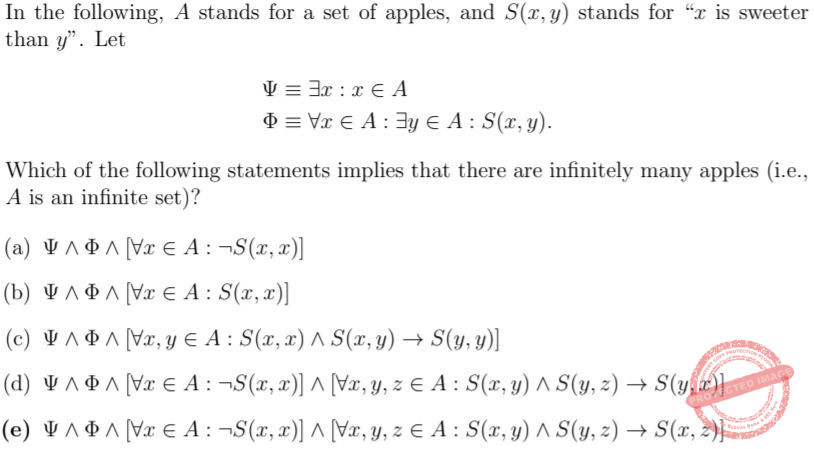A a B b C c D d E e
Engineering-Mathematics       Propositional-Logic
 Question 20
Consider the following recursive function mc91.
int mc91(int n)
{
print n
if (n > 100) { return n - 10;
}
else {
return mc91(mc91(n+11));
}
}
Let
Out = {n : there is an x ∈ {0, 1, . . . , 100} such that n is one of the integers printed by mc91(x)}.
Then, which of the following is Out?
 A {n : −∞ < n ≤ 100} B {n : 0 ≤ n ≤ 101} C {n : 0 ≤ n ≤ 110} D {n : 0 ≤ n ≤ 111} E {n : 0 ≤ n < +∞}
Programming       Functions
 Question 21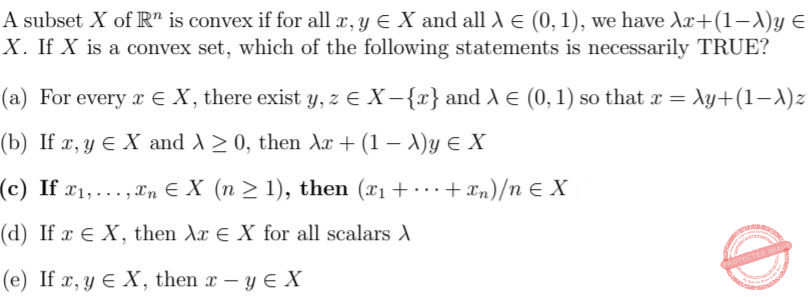A a B b C c D d E e
 Question 22
Let n = m!. Which of the following is TRUE?
 A m = Θ(log n/ log log n) B m = Ω(log n/ log log n) but not m = O(log n/ log log n) C m = Θ(log2 n) D m = Ω(log2 n) but not m = O(log2 n) E m = Θ(log1.5 n)
Algorithms       Asymptotic-Complexity
 Question 23A PRIMES is regular B PRIMES is undecidable C PRIMES is decidable in polynomial time D PRIMES context free but not regular E PRIMES is NP-complete and P ≠ NP
Theory-of-Computation       Languages-and-Grammars
 Question 24
Which of the following graphs DOES NOT have an Eulerian circuit? (Recall that an Eulerian circuit in an undirected graph is a walk in the graph that starts at a vertex and returns to the vertex after travelling on each edge exactly once.)
 A K9,9 B K8,8 C K12,12 D K9 E The graph G on vertex set {1, 2, . . . , 9} with edge set E(G) = {{i, j} : 1 ≤ i < j ≤ 5 or 5 ≤ i < j ≤ 9}.
Engineering-Mathematics       Graph-Theory
 Question 25
A vertex cover in an undirected graph G is a subset C ⊆ V (G) such that every edge of G has an endpoint in C. An independent set in G is a subset I ⊆ V (G) such that no edge has both its endpoints in I. Which of the following is TRUE of every graph G and every vertex cover C of G?
 A There exists an independent set of size |C| B V (G) − C is an independent set C |C| ≥ |E(G)|/2 D |C| ≥ |V (G)|/2 E C intersects every independent set
Engineering-Mathematics       Engineering-Mathematics Graph-Theory
 Question 26A H has an infinite number of vertices B The diameter of H is infinite C H is connected D H contains an infinite clique E H contains an infinite independent set
Engineering-Mathematics       Linear-Algebra
 Question 27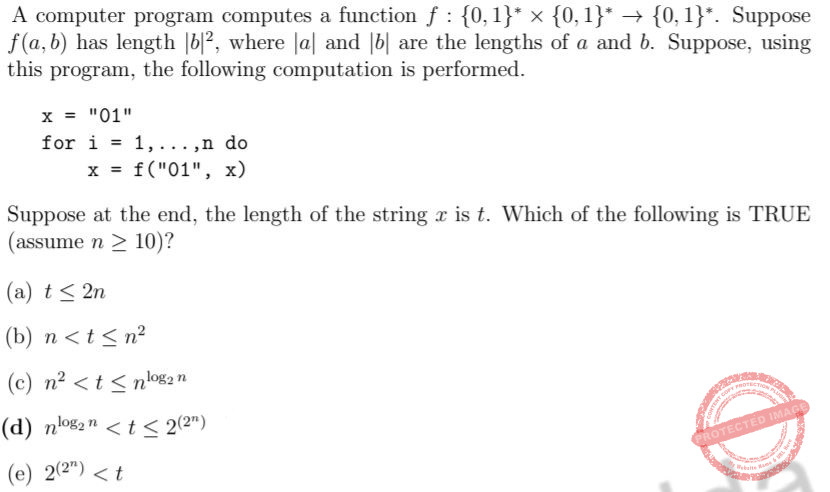A a B b C c D d E e
 Question 28
An undirected graph G = (V, E) is said to be k-colourable if there exists a mapping c : V -->{1, 2, . . . , k} such that for every edge {u, v}ϵE we have c(u) ≠ c(v). Which of the following statements is FALSE?
 A G is |V |-colourable B G is 2-colourable iff there are no odd cycles in G C G is (∆ + 1)-colourable where ∆ is the maximum degree in G D There is a polynomial time algorithm to check if G is 2-colourable E If G has no triangle then it is 3-colourable
Engineering-Mathematics       Graph-Theory
 Question 29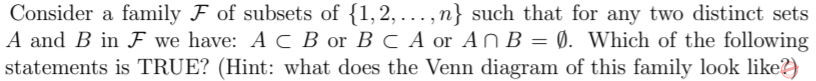A |F| ≤ 2n and there exists a family F such that |F| = 2n B |F| ≤ n2 and there exists a family F such that |F| = n2 C |F| ≤ 2n2 and there exists a family F such that |F| = 2n2 D |F| ≤ 2n−1 and there exists a family F such that |F| = 2n−1 E None of the above
Engineering-Mathematics       Set-Theory
 Question 30
Let G be an undirected graph. For a pair (x, y) of distinct vertices of G, let mincut(x, y) be the least number of edges that should be deleted from G so that the resulting graph has no x-y path. Let a, b, c be three vertices in G such that mincut(a, b) ≤ mincut(b, c) ≤ mincut(c, a). Consider the following possibilities: (i) mincut(a, b) < mincut(b, c) < mincut(c, a) (ii) mincut(a, b) = mincut(b, c) < mincut(c, a) (iii) mincut(a, b) < mincut(b, c) = mincut(c, a) (iv) mincut(a, b) = mincut(b, c) = mincut(c, a) Which of the following is TRUE?
 A All of (i), (ii), (iii), (iv) are possible B (i), (ii), (iii) are possible but not (iv) C (i) and (iv) are possible but neither (ii) nor (iii) D (ii) and (iv) are possible but neither (i) nor (iii) E (iii) and (iv) are possible but neither (i) nor (ii)
Engineering-Mathematics       Graph-Theory
 Question 31A a B b C c D d E e
Engineering-Mathematics       Calculus
 Question 32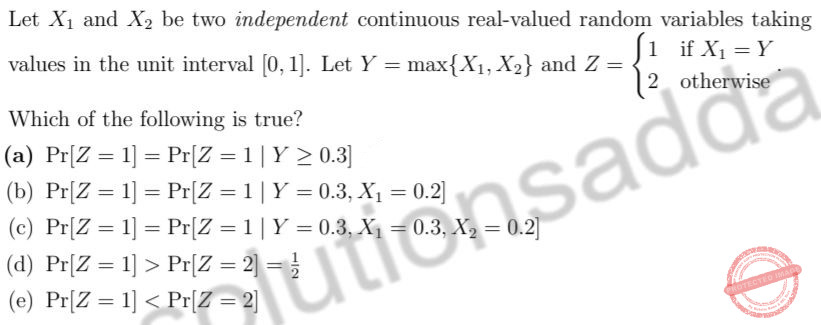A a B b C c D d E e
Engineering-Mathematics       Probability-and-statistics
 Question 33
Let (X, Y ) be a pair of independent random variables. Suppose X takes values in {1, . . . , 6} with equal probability, and Y takes values in {2, 3} with Pr[Y = 2] = p. Let Z = (X mod Y ) + 1.
Which of the statements is true?
 A a B b C c D d E e
Engineering-Mathematics       Probability-and-statistics
 Question 34
Consider a system which in response to input x(t) outputs
y(t) = x(t2).
Which of the following describes the system?
 A linear, time-invariant, causal B linear, time-invariant, non-causal C linear, time-variant D non-linear, time-invariant E non-linear, time-variant
 Question 35A a B b C c D d E e
 Question 36A a B b C c D d E e
Engineering-Mathematics       Calculus
 Question 37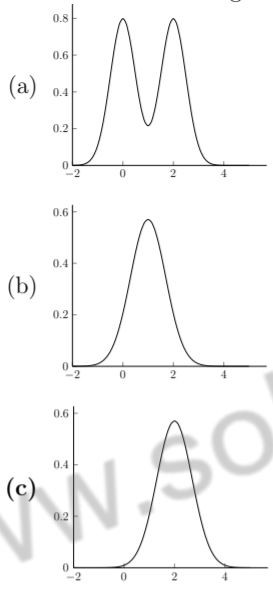A a B v C c D d E e
 Question 38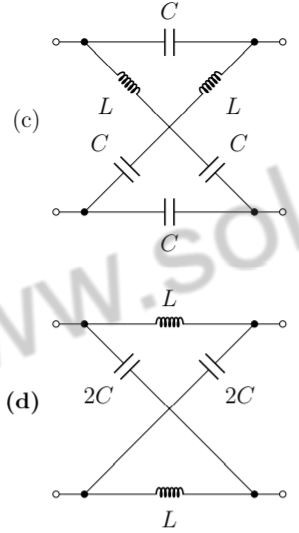A a B b C c D d E All four circuits are equivalent
 Question 39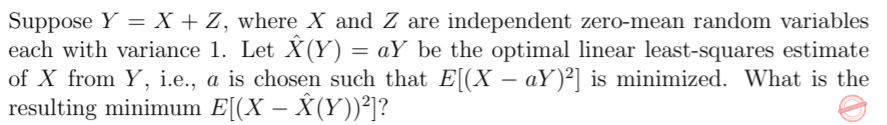A 1 B 2/3 C 1/2 D 1/3 E 1/4
Engineering-Mathematics       Probability-and-statistics
 Question 40
Let U1, U2, U3 be independent random variables that are each uniformly distributed between zero and one. What is the probability that the second highest value amongst the three lies between 1/3 and 2/3?
 A 2/9 B 1/27 C 13/27 D 1/3 E 7/18
Engineering-Mathematics       Probability-and-statistics
 Question 41
Suppose that a random variable X has a probability density function (pdf) given by
f (x) = c exp(−2x)
for x ≥ 1, and f (x) = 0, for x < 1, where c is an appropriate constant so that f (x) is a valid pdf.
What is the expected value of X given that X ≥ 5?
 A 5*(1/2) B 7 C 10 D 8*(1/2) E 6
Engineering-Mathematics       Probability-and-statistics
 Question 42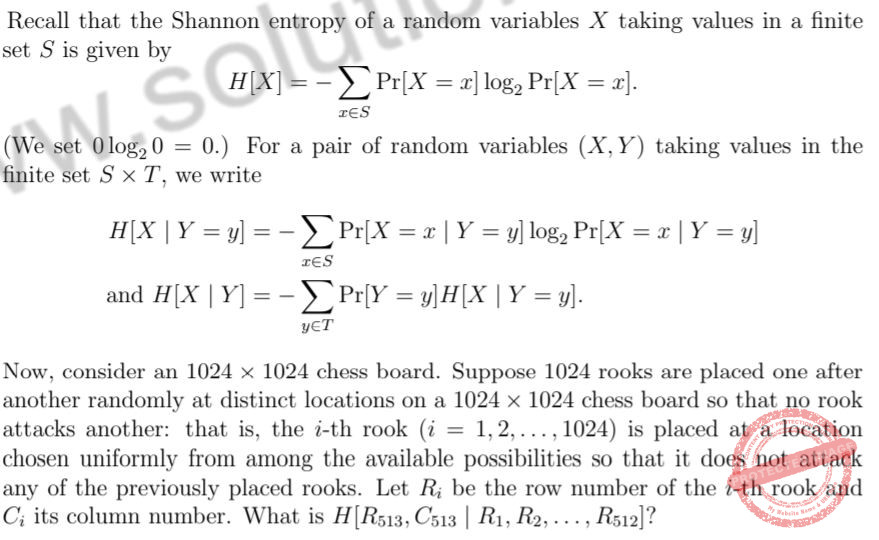A log2513 B 9 C 10 D 19 E 81
 Question 43
Suppose m and n are positive integers, m ≠ n, and A is an m X n matrix with real entries. Consider the following statements.
(i) rank(AAT ) = rank(AT A)
(ii) det(AT A) = det(AAT )
(iii) Trace(AAT ) = Trace(AT A)
Which of the above statements is true for all such A?
 A Only (i) B Only (ii) C Only (iii) D (i) and (iii) E None of them
Engineering-Mathematics       Linear-Algebra
 Question 44A a B b C c D d E e
Engineering-Mathematics       Linear-Algebra
 Question 45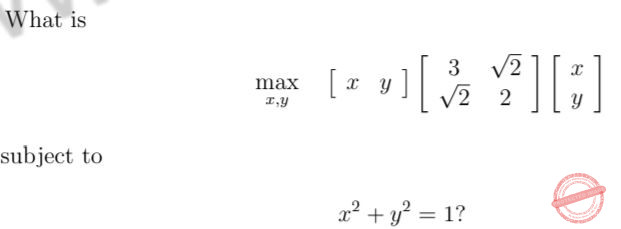A 1 B √2 C 2 D 3 E 4
Engineering-Mathematics       Linear-Algebra
There are 45 questions to complete.